Updating search results...

# 17 Results

View
Selected filters:
• MCCRS.Math.Content.7.EE.A.1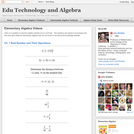Conditional Remix & Share Permitted
CC BY-NC
Rating
0.0 stars

Visually searchable database of Algebra 1 videos. Click on a problem and watch the solution on YouTube. Copy and paste this material into your CMS. Videos accompany the open Elementary Algebra textbook published by Flat World Knowledge.

Subject:
Algebra
Mathematics
Material Type:
Lecture
Lecture Notes
Provider:
Individual Authors
Provider Set:
Individual Authors
Author:
John Redden
04/29/2012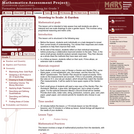Only Sharing Permitted
CC BY-NC-ND
Rating
0.0 stars

This lesson unit is intended to help assess how well students are able to interpret and use scale drawings to plan a garden layout. This involves using proportional reasoning and metric units.

Subject:
Algebra
Geometry
Mathematics
Ratios and Proportions
Material Type:
Assessment
Lesson Plan
Provider:
Shell Center for Mathematical Education
Provider Set:
Mathematics Assessment Project (MAP)
04/26/2013Conditional Remix & Share Permitted
CC BY-NC-SA
Rating
0.0 stars

Students, in partners, will use an online graphing program to complete this discovery lesson. Each pair will change one component of an equation at at time and then complete a worksheet to describe the effect of the change.

Subject:
Mathematics
Material Type:
Activity/Lab
06/20/2016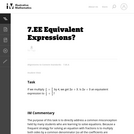Unrestricted Use
CC BY
Rating
0.0 stars

The purpose of this task is to directly address a common misconception held by many students who are learning to solve equations. Because a frequent strategy for solving an equation with fractions is to multiply both sides by a common denominator (so all the coefficients are integers), students often forget why this is an "allowable" move in an equation and try to apply the same strategy when they see an expression.

Subject:
Algebra
Mathematics
Material Type:
Activity/Lab
Provider:
Illustrative Mathematics
Provider Set:
Illustrative Mathematics
Author:
Illustrative Mathematics
05/01/2012Educational Use
Rating
0.0 stars

The CyberSquad tries to figure out how Hackerë_í__ cyberfrog moves when its various buttons are pressed, in this video from Cyberchase.

Subject:
Algebra
Mathematics
Material Type:
Lecture
Provider:
PBS LearningMedia
Provider Set:
PBS Learning Media: Multimedia Resources for the Classroom and Professional Development
Author:
U.S. Department of Education
WNET
07/11/2008Educational Use
Rating
0.0 stars

In this video from Cyberchase, the CyberSquad must figure out the new input/output pattern on Hackerë_í__ larger cyberfrog.

Subject:
Algebra
Mathematics
Material Type:
Lecture
Provider:
PBS LearningMedia
Provider Set:
PBS Learning Media: Multimedia Resources for the Classroom and Professional Development
Author:
U.S. Department of Education
WNET
07/11/2008Educational Use
Rating
0.0 stars

In this activity, students gain first-hand experience with the mechanical advantage of pulleys. Students are given the challenge of helping save a whale by moving it from an aquarium back to its natural habitat into the ocean. They set up different pulley systems, compare the theoretical and actual mechanical advantage of each and discuss their recommendations as a class.

Subject:
Applied Science
Engineering
Physical Science
Physics
Material Type:
Activity/Lab
Provider:
TeachEngineering
Provider Set:
TeachEngineering
Author:
Jake Lewis
Janet Yowell
Malinda Schaefer Zarske
Michael Bendewald
10/14/2015Conditional Remix & Share Permitted
CC BY-NC
Rating
0.0 stars

Four full-year digital course, built from the ground up and fully-aligned to the Common Core State Standards, for 7th grade Mathematics. Created using research-based approaches to teaching and learning, the Open Access Common Core Course for Mathematics&nbsp;is designed with student-centered learning in mind, including activities for students to develop valuable 21st century skills and academic mindset.

Subject:
Mathematics
Material Type:
Full Course
Provider:
Pearson
10/06/2016Conditional Remix & Share Permitted
CC BY-NC
Rating
0.0 stars

Algebraic Reasoning

Type of Unit: Concept

Prior Knowledge

Students should be able to:

Add, subtract, multiply, and divide rational numbers.
Evaluate expressions for a value of a variable.
Use the distributive property to generate equivalent expressions including combining like terms.
Understand solving an equation or inequality as a process of answering a question: which values from a specified set, if any, make the equation or inequality true?
Write and solve equations of the form x+p=q and px=q for cases in which p, q, and x are non-negative rational numbers.
Understand and graph solutions to inequalities x&lt;c or x&gt;c.
Use equations, tables, and graphs to represent the relationship between two variables.
Relate fractions, decimals, and percents.
Solve percent problems included those involving percent of increase or percent of decrease.

Lesson Flow

This unit covers all of the Common Core State Standards for Expressions and Equations in Grade 7. Students extend what they learned in Grade 6 about evaluating expressions and using properties to write equivalent expressions. They write, evaluate, and simplify expressions that now contain both positive and negative rational numbers. They write algebraic expressions for problem situations and discuss how different equivalent expressions can be used to represent different ways of solving the same problem. They make connections between various forms of rational numbers. Students apply what they learned in Grade 6 about solving equations such as x+2=6 or 3x=12 to solving equations such as 3x+6=12 and 3(x−2)=12. Students solve these equations using formal algebraic methods. The numbers in these equations can now be rational numbers. They use estimation and mental math to estimate solutions. They learn how solving linear inequalities differs from solving linear equations and then they solve and graph linear inequalities such as −3x+4&lt;12. Students use inequalities to solve real-world problems, solving the problem first by arithmetic and then by writing and solving an inequality. They see that the solution of the algebraic inequality may differ from the solution to the problem.

Subject:
Algebra
Mathematics
Material Type:
Unit of Study
Provider:
PearsonConditional Remix & Share Permitted
CC BY-NC
Rating
0.0 stars

Students match equations such as 3x &minus; 50 = 90 and 3(x &minus; 50) = 90 to real-world and mathematical situations. They identify the steps needed to solve these equations.Key ConceptsStudents solve equations such as 3x &minus; 50 = 90 by using first the addition property and then the multiplication property of equality.Students also solve equations such as 3(x &minus; 50) = 90. Equations with parentheses were introduced in the Challenge Problem of Lesson 6. Now, in this lesson, students use two methods to solve the equation. First method: use the multiplication property of equality and then the addition property of equality; second method: use the distributive property to eliminate the parentheses, then use the addition property of equality, and then the multiplication property of equality.Goals and Learning ObjectivesMatch equations to problems.Solve two-step equations.

Subject:
Algebra
Material Type:
Lesson Plan
09/21/2015Conditional Remix & Share Permitted
CC BY-NC
Rating
0.0 stars

Students use the distributive property to simplify expressions. Simplifying expressions may include multiplying by a negative number. Students analyze and identify errors that are sometimes made when simplifying expressions.Key ConceptsThis lesson focuses on simplifying expressions and requires an understanding of the rules for multiplying negative numbers. For example, students simplify expressions such as 8 &minus; 3(2 &minus; 4x). These kinds of expressions are often difficult for students because there are several errors that they can make based on misconceptions:Students may simplify 8 &minus; 3(2 &minus; 4x) to 5(2 &minus; 4x) because they mistakenly detach the 3 from the multiplication.Students may simplify 8 &minus; 3(2 &minus; 4x) to 8 &minus; 3(&minus;2x) in an attempt to simplify the expression in parentheses even though no simplification is possible.Students may simplify 8 &minus; 3(2 &minus; 4x) to 8 &minus; 6 &minus;12x. This error could be based on a misunderstanding of how the distributive property works or on lack of knowledge of the rules for multiplying integers.Goals and Learning ObjectivesSimplify more complicated expressions that involve multiplication by negative numbers.Identify errors that can be made when simplifying expressions.

Subject:
Algebra
Material Type:
Lesson Plan
09/21/2015Conditional Remix & Share Permitted
CC BY-NC
Rating
0.0 stars

Students discover how the addition and multiplication properties of inequality differ from the addition and multiplication properties of equality.Students use the addition and multiplication properties of inequality to solve inequalities. They graph their solutions on the number line.Key ConceptsIn this lesson, students extend their knowledge of inequalities from Grade 6. In Grade 6, students learned that solving an inequality meant finding which values made the inequality true. Students used substitution to determine whether a given value made an inequality true. They also used a number line to graph the solutions of inequalities. By graphing these solutions on a number line, they saw that an inequality has an infinite number of solutions.Now, in Grade 7, students work with inequalities that also contain negative numbers and learn to solve and graph solutions for inequalities such as &minus;2x &minus; 4 &lt; 5. This involves first understanding how the properties of inequality differ from the properties of equality. When multiplying (or dividing) both sides of an inequality by the same negative number, the relationship between the two sides of the inequality changes, so it is necessary to reverse the direction of the inequality sign in order for the inequality to remain true. Once students understand this, they can apply the same steps they used to solve equations to solve inequalities, but remembering to reverse the direction of the inequality sign when multiplying or dividing both sides of the inequality by a negative number.Goals and Learning ObjectivesAccess prior knowledge of how to solve an inequality.Observe that when multiplying or dividing both sides of an inequality by the same negative number, the inequality sign must change direction.Solve and graph inequalities of the form px + q &gt; r or px + q &lt; r, where p, q, and r are specific rational numbers.

Subject:
Algebra
Material Type:
Lesson Plan
09/21/2015Conditional Remix & Share Permitted
CC BY-NC
Rating
0.0 stars

Students extend what they learned about solving equations in Grade 6. They learn to solve equations that require them to use both the addition and the multiplication properties of equality. They use what they know about solving equations such as 2x = 6 and x + 3 = 7 to solve equations such as 2x + 3 = 8. They connect solving problems using arithmetic to solving problems using equations. They solve equations containing both positive and negative rational numbers.Key ConceptsAddition property of equality: If a = b, then a + c = b + c.Multiplication property of equality: If a = b, then ac = bc.For any equation, add or subtract the same value from both sides of the equation and the equation will still be true.For any equation, multiply or divide both sides of the equation by the same value and the equation will still be true.In this lesson, students use both properties to solve equations. They then solve equations that contain both positive and negative rational numbers.Goals and Learning ObjectivesSolve equations using both the addition and multiplication properties of equality.Relate solving problems using arithmetic to solving problems using equations.Solve equations containing both positive and negative rational numbers.

Subject:
Algebra
Material Type:
Lesson Plan
09/21/2015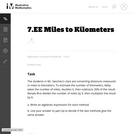Unrestricted Use
CC BY
Rating
0.0 stars

In this task students are asked to write two expressions from verbal descriptions and determine if they are equivalent. The expressions involve both percent and fractions. This task is most appropriate for a classroom discussion since the statement of the problem has some ambiguity.

Subject:
Algebra
Mathematics
Material Type:
Activity/Lab
Provider:
Illustrative Mathematics
Provider Set:
Illustrative Mathematics
Author:
Illustrative Mathematics
05/01/2012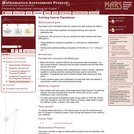Only Sharing Permitted
CC BY-NC-ND
Rating
0.0 stars

This lesson unit is intended to help teachers assess how well students are able to: form and solve linear equations involving factorizing and using the distributive law. In particular, this unit aims to help teachers identify and assist students who have difficulties in: using variables to represent quantities in a real-world or mathematical problem and solving word problems leading to equations of the form px + q = r and p(x + q) = r.

Subject:
Algebra
Mathematics
Material Type:
Assessment
Lesson Plan
Provider:
Shell Center for Mathematical Education
Provider Set:
Mathematics Assessment Project (MAP)
04/26/2013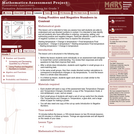Only Sharing Permitted
CC BY-NC-ND
Rating
0.0 stars

This lesson unit is intended to help teachers assess how well students are able to understand and use directed numbers in context. It is intended to help identify and aid students who have difficulties in ordering, comparing, adding, and subtracting positive and negative integers. Particular attention is paid to the use of negative numbers on number lines to explore the structures: starting temperature + change in temperature = final temperature final temperature Đ change in temperature = starting temperature final temperature Đ starting temperature = change in temperature.

Subject:
Mathematics
Numbers and Operations
Material Type:
Assessment
Lesson Plan
Provider:
Shell Center for Mathematical Education
Provider Set:
Mathematics Assessment Project (MAP)
04/26/2013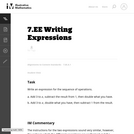Unrestricted Use
CC BY
Rating
0.0 stars

The instructions for the two expressions sound very similar, however, the order in which the different operations are performed and the exact wording make a big difference in the final expression. Students have to pay close attention to the wording: Ňsubtract the result from 1Ó and Ňsubtract 1 from the resultÓ are very different.

Subject:
Mathematics
Material Type:
Activity/Lab
Provider:
Illustrative Mathematics
Provider Set:
Illustrative Mathematics
Author:
Illustrative Mathematics